The program mcfluor.c is a C program that will call the subroutine mcsubLIB.c, which executes Monte Carlo simulations using the parameters supplied by mcfluor.c.

The program is basically the same as published in Jacques SL, Monte Carlo simulations of fluorescence in turbid media, Ch. 6 in Handbook of Biomedical Fluorescence, M.A. Mycek, B.W. Pogue, publ. Marcel-Dekker, New York, NY, 2003. There are some minor changes, some because the mcsubLIB.c has been updated, for example, to allow for a mismatched boundary condition or to launch a beam at a subsurface location (to simulate a submerged optical fiber), and some changes cleaning up the code.

The run parameters are selected in the program. In the example shown here, the product of extinction coefficient and concentration is eC = 1 cm-1 = (cm-1 M-1)(M), and the energy yield is Y = 1 = 1 [J fluorescence per J excitation absorbed by fluorophore]. The results can be scaled by the actual values of eC and Y. To compile the program for execution, one simply types:

`cc -o gomcsub mcsubLIB.c mcfluor.c`

that yields the executable gomcsub*. Sometimes one might need to type

`cc -lm -o gomcsub mcsubLIB.c mcfluor.c`

to help the compiler find the math library. The details of compilation using your compiler may differ slightly. Once compiled, simply typing ./gomcsub on a Unix machine will execute the program from within the same directory where the program resides. Again, calling the program differs with the operating system being used.

The program files are

The output files are

1. mcOUT101.dat = excitation files
2. mcOUT102.dat = emission files for background uniform fluorescence
3. mcOUT103.dat = emission files for positive-x half of asymmetric heterogeneous source
4. mcOUT104.dat = emission files for negative-x half of asymmetric heterogeneous source

A MATLAB program will read these files and create the figures 1 through 6 shown below.
lookmcsubfluor.m creates the figures, and uses the following routines:
makec2f.m creates a colormap used in plotting.

Example Run
Running the compiled C program yields the 4 output files and the following printout on the console. This was a short run (controlled by selecting Nruns = 0.1) which only required 1.3 min to complete, so the data is a little noisy. The MATLAB-generated figures from the output files are shown, then the printout is listed after the figures.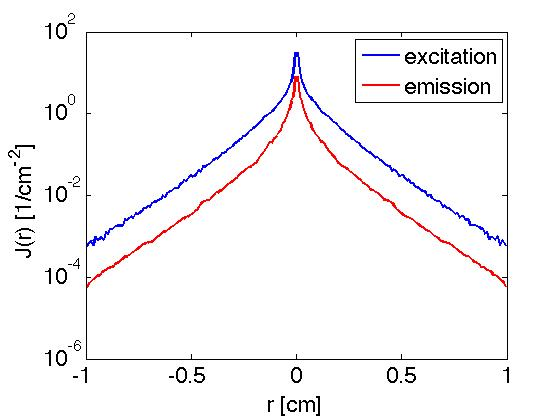Fig. 1: Escaping fluxes Ex and Em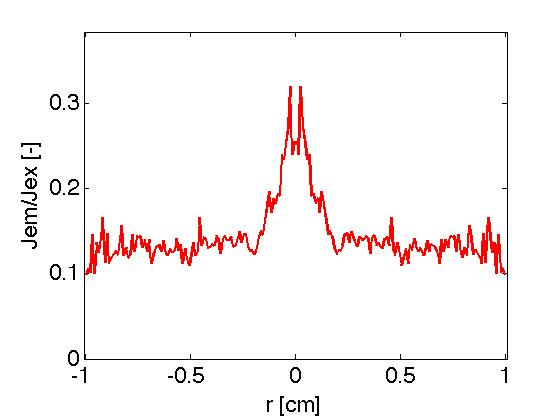Fig. 2: Ratio of escaping Ex/Em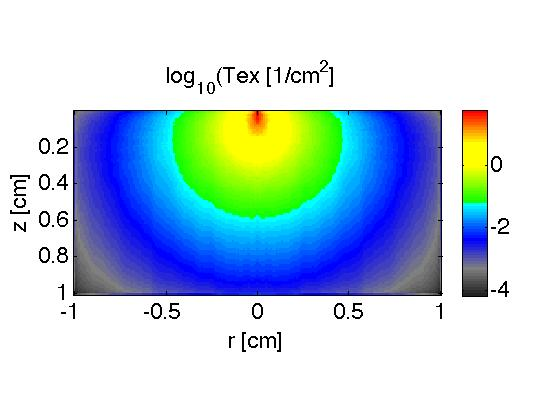Fig. 3: Excitation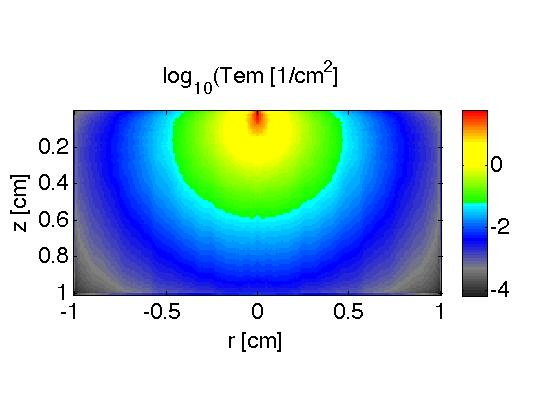Fig. 4: Background fluorescence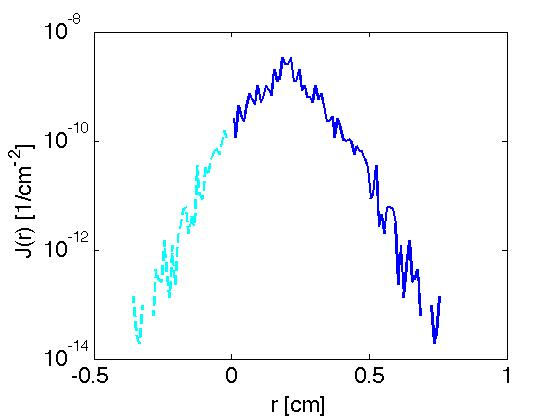Fig. 5: Off-center local fluorophore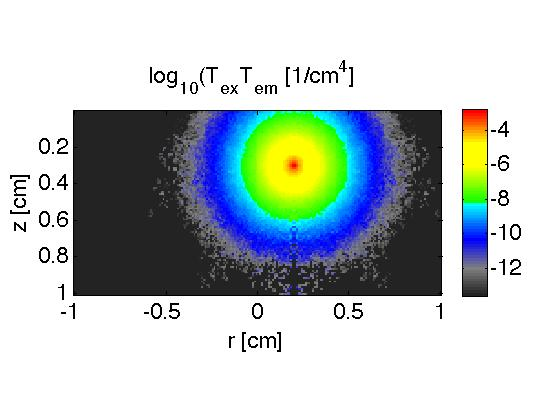Fig. 6: Off-center local fluorophore

Output to console from example run

```\$ gomcsub
Wed Oct 24 22:19:30 2007

----- USER CHOICES -----
EXCITATION
muax = 1.000
musx = 100.000
gx = 0.900
n1 = 1.330
n2 = 1.000
mcflag = 0
waist = 0.0000
zfocus = 0.0000
xs = 0.0000
ys = 0.0000
zs = 0.0000
BACKGROUND FLUORESCENCE
muaf = 5.000
musf = 50.000
gf = 0.000
eC = 1.000
Y = 1.000
FLUORESCENT HETEROGENEITY
xh = 0.2000
yh = 0.0000
zh = 0.3000
heC = 0.1000
hY = 1.0000
OTHER
Nruns = 0.1 @1e6 photons/run
dr = 0.0100
dz = 0.0100
---------------

3.504e-06 min/EX photon
8.342e-07 min/EM photon

Total estimated completion time =  1.28 min

EXCITATION
est. completion time =  0.35 min

100     photons
200     photons
300     photons
400     photons
500     photons
600     photons
700     photons
800     photons
900     photons
1000     photons
2000     photons
3000     photons
4000     photons
5000     photons
6000     photons
7000     photons
8000     photons
9000     photons
10000    photons
20000    photons
30000    photons
40000    photons
50000    photons
60000    photons
70000    photons
80000    photons
90000    photons
100000   photons
------------------------------------------------------
------------------------------------------------------
Elapsed Time for excitation = 0.361 min
Wed Oct 24 22:19:52 2007

BACKGROUND FLUORESCENCE
est. completion time =  0.85 min

10000 fluor photons     @ ir = 1         total = 10000
10000 fluor photons     @ ir = 2         total = 20000
10000 fluor photons     @ ir = 3         total = 30000
10000 fluor photons     @ ir = 4         total = 40000
10000 fluor photons     @ ir = 5         total = 50000
10000 fluor photons     @ ir = 6         total = 60000
10000 fluor photons     @ ir = 7         total = 70000
10000 fluor photons     @ ir = 8         total = 80000
10000 fluor photons     @ ir = 9         total = 90000
10000 fluor photons     @ ir = 10        total = 100000
10000 fluor photons     @ ir = 20        total = 200000
10000 fluor photons     @ ir = 30        total = 300000
10000 fluor photons     @ ir = 40        total = 400000
10000 fluor photons     @ ir = 50        total = 500000
10000 fluor photons     @ ir = 60        total = 600000
10000 fluor photons     @ ir = 70        total = 700000
10000 fluor photons     @ ir = 80        total = 800000
10000 fluor photons     @ ir = 90        total = 900000
10000 fluor photons     @ ir = 100       total = 1000000
10000 fluor photons     @ ir = 101       total = 1000000
1000000 fluorescent photons total
Elapsed Time for background fluorescence = 0.863 min
------------------------------------------------------
FLUORESCENT HETEROGENEITY
est. completion time = 0.083 min

100     photons
200     photons
300     photons
400     photons
500     photons
600     photons
700     photons
800     photons
900     photons
1000     photons
2000     photons
3000     photons
4000     photons
5000     photons
6000     photons
7000     photons
8000     photons
9000     photons
10000    photons
20000    photons
30000    photons
40000    photons
50000    photons
60000    photons
70000    photons
80000    photons
90000    photons
100000   photons
Elapsed Time for fluorescent heterogeneity = 0.084 min
------------------------------------------------------
------------------------------------------------------
Wed Oct 24 22:20:49 2007

Estimated total completion time = 1.28 min

Actual total elapsed time = 1.31 min
\$

```